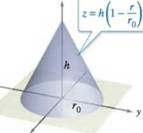Chapter 14.7, Problem 27E

Chapter
Section
Textbook Problem

Using Cylindrical Coordinates In Exercises 23-28, use cylindrical coordinates to find the indicated characteristic of the cone shown in the figure.Assume that the cone has uniform density and show that the moment of inertia about the z-axis is I z = 3 10 m r 0 2

To determine

To prove: The moment of inertia with respect to the z-axis of provided cone is Iz=310mr02

Explanation

Given:

The cylindrical coordinates of the cone indicated by the equation z=h(1rr0) is represented in the following figure

Formula Used:

The moments are found by using the formula:

Myz=Qxρ(x,y,z)dVMxz=Qyρ(x,y,z)dVMxy=Qzρ(x,y,z)dV

And the formula of integration:

xndx=xn+1n+1

Proof:

The moment of inertia about z-axis is represented by

Iz=R(x2+y2)ρ(x,y,z)dV

By substituting the values and formula,

xndx=xn+1n+1

It is found that

Mxy=4k0π20r00h(r0r)r0</

Still sussing out bartleby?

Check out a sample textbook solution.

See a sample solution

The Solution to Your Study Problems

Bartleby provides explanations to thousands of textbook problems written by our experts, many with advanced degrees!

Get Started

Find more solutions based on key concepts